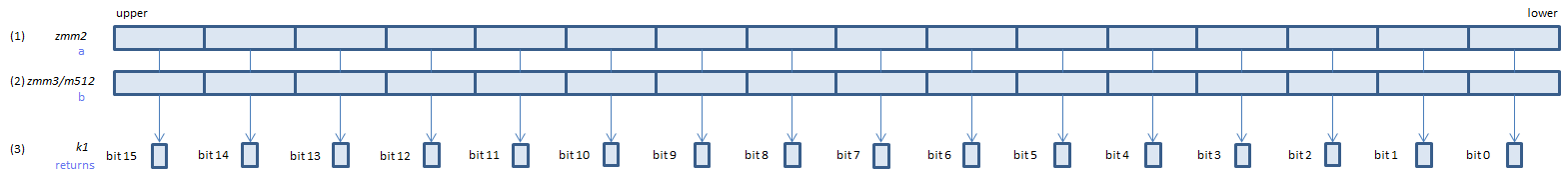﻿ vcmpge_oqps

## VCMPGE_OQPS - CoMPare Greater Equal Ordered Quiet Packed Single

VCMPGE_OQPS xmm1, xmm2, xmm3/m128    (V1
__m128 _mm_cmp_ps(__m128 a, __m128 b, _CMP_GE_OQ)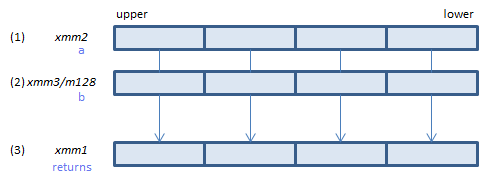For each float, if (1) >= (2) set 1, else set 0, to all bits of the corresponding float of (3).
VCMPGE_OQPS ymm1, ymm2, ymm3/m256    (V1
__m256 _mm256_cmp_ps(__m256 a, __m256 b, _CMP_GE_OQ)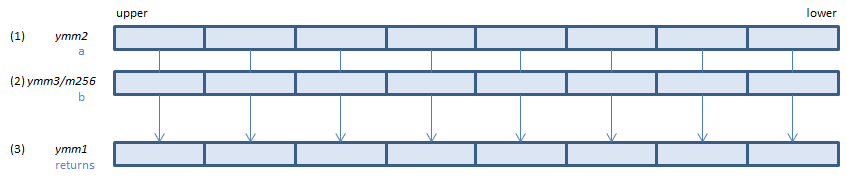For each float, if (1) >= (2) set 1, else set 0, to all bits of the corresponding float of (3).
VCMPGE_OQPS k1{k2}, xmm2, xmm3/m128/m32bcst    (V5+VL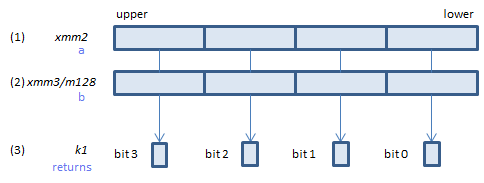For each float, if (1) >= (2) set 1, else set 0, to the corresponding bit of (3).
If k2 bit is 0, the comparison is not done and the corresponding bit of (3) is set to zero. Upper bits of (3) are zero cleared.
VCMPGE_OQPS k1{k2}, ymm2, ymm3/m256/m32bcst    (V5+VL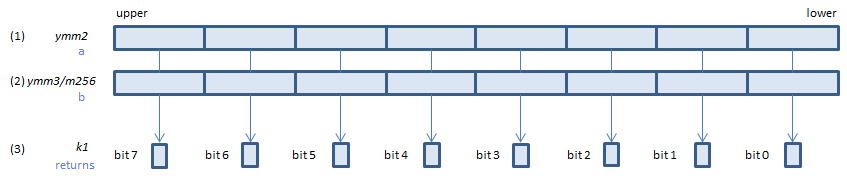For each float, if (1) >= (2) set 1, else set 0, to the corresponding bit of (3).
If k2 bit is 0, the comparison is not done and the corresponding bit of (3) is set to zero. Upper bits of (3) are zero cleared.
VCMPGE_OQPS k1{k2}, zmm2, zmm3/m512/m32bcst{sae}    (V5